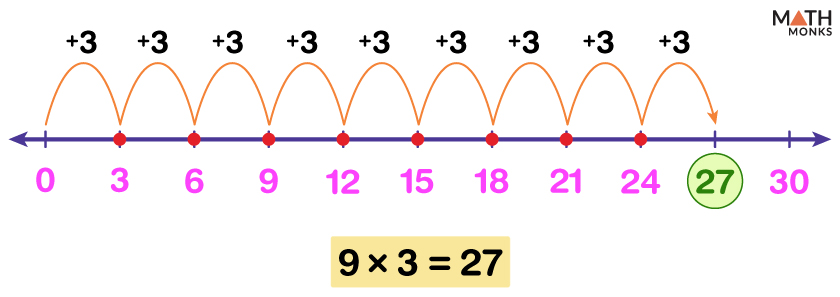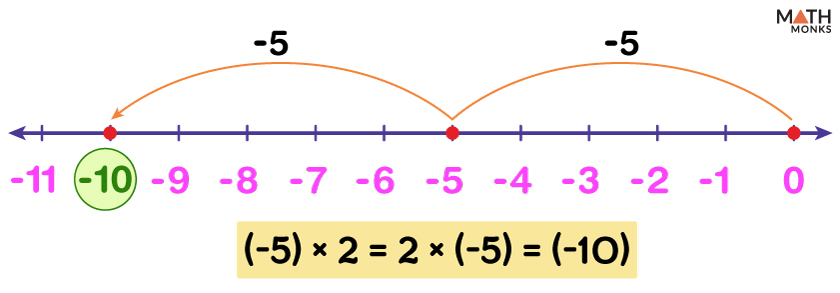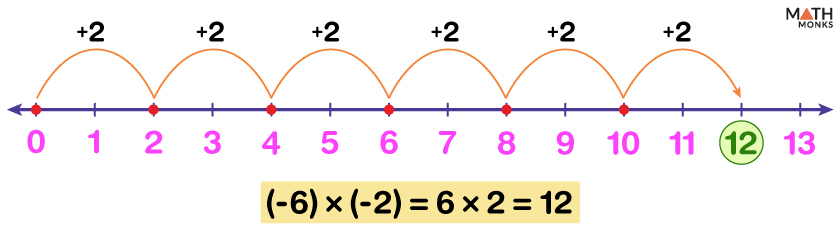# Multiplication on a Number Line

Multiplication on a number line is performing the multiplication operation on a set of numbers and finding the result using a number line.

Since multiplication is also known as repeated addition, the result is obtained after performing addition for the required number of times.

Using a number line, we can multiply two positive numbers, two negative numbers, or a positive and a negative number.

## With Positive Numbers

While multiplying two positive numbers, we move towards the right on a number line, starting from 0.

For example, 3 × 4 represents 3 times 4 or 3 groups of 4.

Step 1: 4 being positive, we move 4 units to the right of 0.

Step 2: A total of 3 such moves are taken, i.e., we skip count forward by 4s three times to the right of 0.

Thus, we get 3 × 4 = 12 on the number line.

Find 9 × 3 using a number line.

Solution:As 9 × 3 means 9 times 3, the result is obtained by adding 3 nine times.
At first, a number line with the first few positive multiples of 3 is drawn. Then we skip count by 3s to the right starting from 0 nine times.
Thus we find 9 × 3 = 27 on the number line.

## With a Positive and a Negative Number

Multiplicating a positive and a negative number gives a negative number by the odd rule of multiplying negative numbers.

Let us multiply 6 × (-4)

6 × (-4) means 6 times of (-4).Thus we need to add (-4) six times.

Step 1: As (-4) is negative and lies to the left of 0, a negative number line is drawn by plotting the first few negative multiples of 4 starting from 0.

Step 2: Then, we count back by 4s six times to the left, starting from 0.

Thus we locate the result 6 × (-4) = (-24) on the number line.

Calculate (-5) × 2 on a number line.

Solution:As we know, multiplication is commutative; we can write (-5) × 2 as 2 × (-5), which means 2 times (-5).
Here the result is obtained by adding (-5) two times, i.e., 2 × (-5) = (-5) + (-5)
Thus counting back by 5s twice to the left, starting from 0, will get us to -10.
So the product is (-5) × 2 = 2 × (-5) = (-10) is on the number line.

## With Negative Numbers

Multiplication of two negative numbers gives a positive number by the even rule of multiplying negative numbers.

For instance, to multiply (-7) × (-4), we see that the result will be positive as both the numbers are negative.

So we calculate 7 × 4 following the rule of multiplying positive numbers.

Step 1: A positive number line starting at 0 and with the first few multiples of 4 is drawn.

Step 2: As 7 × 4 denotes 7 times 4, we count forward by 4s seven times to the right starting from 0.

Thus we get the result (-7) × (-4) = 7 × 4 = 28

Find the product (-6) × (-2) using a number line.

Solution:This multiplication result will be positive as (-6) and (-2) are negative numbers.
Thus (-6) × (-2) can be written as 6 × 2, i.e., 6 times 2.
To add 2 eight times, we count forward by 2s six times to the right starting from 0.
We find (-6) × (-2) = 6 × 2 = 12.

## Multiplication of Fractions

A number line can also be used to multiply fractions by integers or another fraction and find the result.

For example, ${\dfrac{1}{5}}$ × ${\dfrac{2}{3}}$, can be written as ${\dfrac{1}{5}}$ of ${\dfrac{2}{3}}$.

Step 1: As the second fraction ${\dfrac{2}{3}}$ lies between 0 and 1, the region from 0 to 1 is divided into 3 equal parts.

Each of the two parts from 0 to ${\dfrac{1}{3}}$ and from ${\dfrac{1}{3}}$ to ${\dfrac{2}{3}}$ is highlighted.

Step 2: The denominator of the first fraction ${\dfrac{1}{5}}$ is 5, so each of the 3 parts is again subdivided into 5 equal parts.

Thus there is a total of 15 parts from 0 to 1.

Step 3: Since the numerator of the first fraction ${\dfrac{1}{5}}$ is 1, we highlight the 1st part of each subdivided part from 0 to ${\dfrac{2}{3}}$.

This is ${\dfrac{1}{5}}$ of the two third or ${\dfrac{1}{5}}$ of ${\dfrac{2}{3}}$

Step 4: As there is a total of 15 parts, the first fraction between 0 and ${\dfrac{1}{3}}$ can be written as ${\dfrac{1}{15}}$ or 1 part of total 15 parts.

Similarly, the first fraction between ${\dfrac{1}{3}}$ and ${\dfrac{2}{3}}$ is written as ${\dfrac{1}{15}}$ or 1 part of total 15 parts.

Adding these two fractions, we get the result  ${\dfrac{1}{15}}$ +  ${\dfrac{1}{15}}$ = ${\dfrac{2}{15}}$ So, ${\dfrac{1}{5}}$ × ${\dfrac{2}{3}}$ =  ${\dfrac{2}{15}}$.